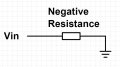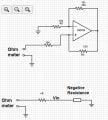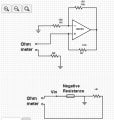# What are the applications of negative resistance?

#### Michael George

Joined Feb 8, 2015
53
I read this site and built negative resistance using opamp.How Can I test this circuit? I'm not able to measure it using an ordinary multimeter. What is the most simple application or project that I can build and test this phenomenon?

I have a second question. I know that the oscillations of LC circuit decay due to resistance. Can I use negative resistance to cancel the positive resistance of LC circuit and make an oscillator?

Thank you,

#### ErnieM

Joined Apr 24, 2011
8,355
1. Put a known resistor in series with the negative resistance. As long as the positive resistance is greater than the negative resistance you should be able to read the difference on an ohmmeter.

2. Probably not, unless you are adept at balancing a pencil on a well sharpened point. Blindfolded and wearing mittens. You need to counteract the resistance exactly or the oscillation stops, or grows and the output saturates.

•Michael George

#### Papabravo

Joined Feb 24, 2006
20,147
For further research and applications look up "tunnel diode".

•cmartinez and Michael George

#### Michael George

Joined Feb 8, 2015
53
in series
A normal resistance has two terminals. But the circuit of negative resistance has four terminals (Vcc , Ground , Vin , Vout). Where are the two terminals of the negative resistance? so that I can connect a series positive resistance?
I can not connect a series positive resistance because I don't know what the two terminals of the negative resistance circuit are.

#### ErnieM

Joined Apr 24, 2011
8,355
The two terminals are as marked on your drawing: Vin and ground.

#### Michael George

Joined Feb 8, 2015
53
@ErnieM Hello againThe two terminals are as marked on your drawing: Vin and ground.
Upon your answer, I think the negative resistance circuit can be represented by the following figure:Now, There are two cases to connect a positive series resistoance:
The first case is to connect one terminal of the positive resistance to the Vin terminal. Now, the Vin terminal is the common terminal between the two resistors. And I should connect the two terminals of the ohmmeter to the ground and the other terminal of the positive resistor as shown in figure:The second case is that the ground will be the common terminal between the two resistors. As shown in the following figure:The problem is that the ohmmeter measure about 1K ohm in both cases no matter the value of the positive resistor. I mean the measured value is not the difference between the positive and negative resistors, It is always 1K.

Also, When I change the "scale" of the ohmmeter, The measured value changed to about 41K and it also does not respond to the value of the positive resistor.

I tried to put connect them in parallel but the ohmmeter measures wrong values.

Would you tell me what I'm missing, Please? or Would you give me another way to test the circuit,Please?

Thank you very much.

#### Picbuster

Joined Dec 2, 2013
1,041
I read this site and built negative resistance using opamp.How Can I test this circuit? I'm not able to measure it using an ordinary multimeter. What is the most simple application or project that I can build and test this phenomenon?

I have a second question. I know that the oscillations of LC circuit decay due to resistance. Can I use negative resistance to cancel the positive resistance of LC circuit and make an oscillator?

Thank you,
Yes, a negative impedance is mandatory for an oscillator to run (based on a feedback mechanism).

•Michael George

#### DickCappels

Joined Aug 21, 2008
9,871
One practical use of negative resistance was with a cheap hobby drill that I once bought. With a constant voltage power supply, the motor would slow down when under load, which made it difficult to guide the tool when routing. Now I power the drill from a power supply with a negative output resistance that partially cancels the resistance in the armature and lead wires so that now the drill maintains nearly constant speed under load and operates much more smoothly than before.

Similarly, speaker amplifiers with negative output impedance can cancel the impedance of the speaker to greatly improve damping.

Another use is to drive a current transformer with a negative resistance to cancel the resistance of the output winding. The result can dramatically reduce the low frequency cutoff, to nearly what one would call DC.

#### ErnieM

Joined Apr 24, 2011
8,355
You might try applying a test voltage, say 1V, and measure the voltage across R4.

•Michael George

#### hp1729

Joined Nov 23, 2015
2,304
I read this site and built negative resistance using opamp.How Can I test this circuit? I'm not able to measure it using an ordinary multimeter. What is the most simple application or project that I can build and test this phenomenon?

I have a second question. I know that the oscillations of LC circuit decay due to resistance. Can I use negative resistance to cancel the positive resistance of LC circuit and make an oscillator?

Thank you,
The 4250 op amp has a load of 200 ohms. Connecting it to a circuit that has a negative 200 ohms input resistance results in "effectively infinite load resistance" (it says).
For testing negative resistance how about something like Design 636. I haven't tried it yet.

#### Attachments

• 6.6 KB Views: 8
• 38.4 KB Views: 5
Last edited:
•Michael George

#### DickCappels

Joined Aug 21, 2008
9,871
Not exactly an infinite load resistance. To wit:

200Ω - 200Ω = 0Ω

•Michael George

#### hp1729

Joined Nov 23, 2015
2,304
Not exactly an infinite load resistance. To wit:

200Ω - 200Ω = 0Ω
???? +1 mA and - 1 mA = 0 mA = infinite ohms
I am still trying to make sense of the article he references. The article uses op amps as an example. The input resistance (input current it looks like to me) of an op amp to cancel the load resistance on the output of another op amp. At the output of the op amp you have load current "cancelled" by an equal and opposite current from the input of another op amp. ???

#### DickCappels

Joined Aug 21, 2008
9,871
It is the other way around if it is a negative resistance circuit (and it looks like it). With a 200 Ω load and a negative resistance 200 Ω load (-200Ω) the sum of the resistances would be zero.

I've done it in a couple of circuits and it really works that way.

#### MrChips

Joined Oct 2, 2009
28,511
I concur with Dick. Negative resistance is used in motor speed control and electronic brakes. I used a negative resistance amplifier to start/stop DC motors in the days of digital recording on audio-cassette recorders. The motor speed is proportional to the input voltage to the amplifier. When 0V is applied, the motor stopped dead in its tracks.

#### hp1729

Joined Nov 23, 2015
2,304
Yes, this means that all the current is provided via second op amp.
View attachment 100824
??? Did I do something wrong in my circuit. I can't make sense of my results.
I didn't measure the driving current inside the feedback loop. Corrected schematic attached.

#### Attachments

• 40.8 KB Views: 10
Last edited:

#### Jony130

Joined Feb 17, 2009
5,445

#### ErnieM

Joined Apr 24, 2011
8,355
The physical 200 ohm resistor and the op amp negative 200 ohm resistor are not in series. They are in parallel.

Good luck figuring out the parallel resistance as ohms law does not apply when dividing by zero.

#### DickCappels

Joined Aug 21, 2008
9,871
The physical 200 ohm resistor and the op amp negative 200 ohm resistor are not in series. They are in parallel.

Good luck figuring out the parallel resistance as ohms law does not apply when dividing by zero.
That is exactly the problem I experienced in one application. When they are in parallel and the positive and negative resistances become equal to one-another, the gain approaches infinity and the circuit latches up!

In the case of parallel impedances, such as when terminating a current transformer, you can get very close to zero ohms but the moment you approach zero from either side, all bets are off.Approaching from the left, the impedance tends toward negative infinity just as approaching from the right the impedance tends toward positive infinity. In theory, at the point they are equal and opposite, the resistance at the input is equal parts +infinite ohms and –infinite ohms and the series resistance is zero ohms.

#### ErnieM

Joined Apr 24, 2011
8,355
??? Did I do something wrong in my circuit. I can't make sense of my results.